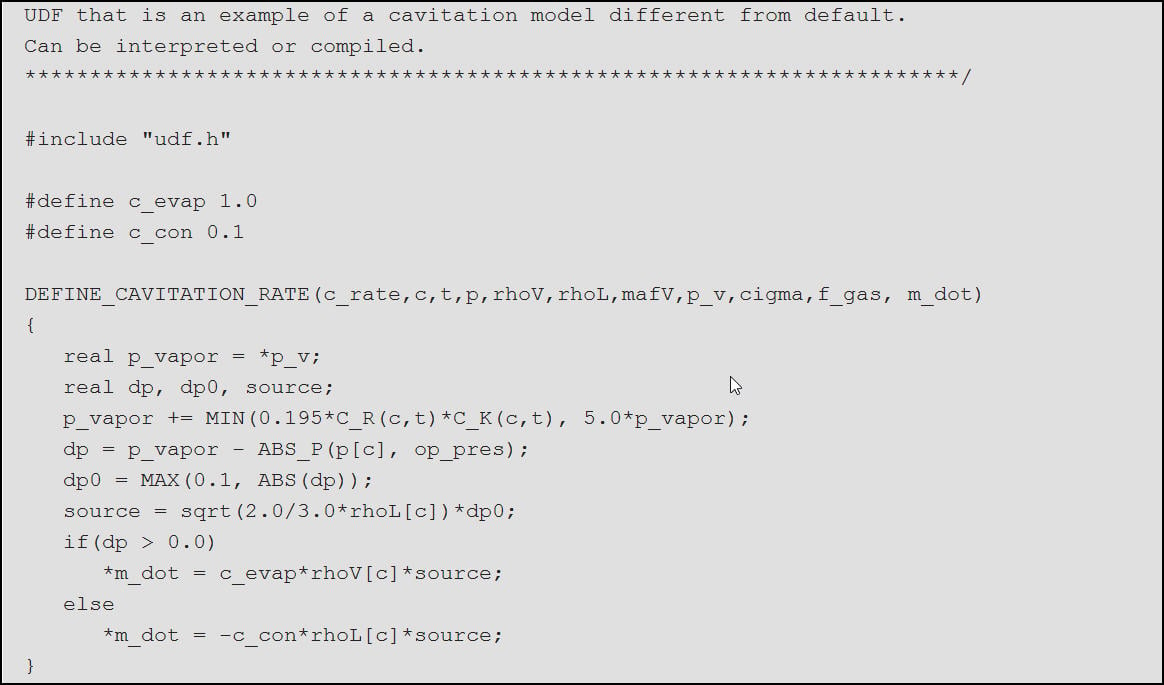## Fluids

•ledinhanh
Subscriber

Hi,

I am making the UDF cavitation based on Merkle cavitation model.

The UDF base on the manual provided by Ansys Fluent 12.0. In that, they states as: "Assuming mdot denotes the mass transfer rate between liquid and vapor phases, we have Re = MAX[mdot, 0]fl Rc = MAX[−mdot, 0]fv where fl and fv are the mass-fraction of the liquid and vapor phase, respectively. DEFINE CAVITATION RATE is used to calculate mdot only. The values of Re and Rc are computed by the solver, accordingly."

It means that when calculation mdot, I need to divide the mdot by fl for evaporation, and by fv for condensation; then the solver will calculate the Re and Rc automatically by multiple with fl and fv respectively to return the correct value ???

Can you help me correct it???

•Keyur Kanade
Ansys Employee

fl and fv are the mass-fraction of the liquid and vapor phase, respectively.

DEFINE_CAVITATION_RATE is used to calculate  only. The values of Re and Rc are computed by the solver, accordingly.

An example of udf is below.

Regards,

Keyur•ledinhanh
Subscriber

Thank Keyur,

I known this example. My question is forexample, in Schneer-Sauer modelso, to calculate *mdot, I need divide Re by fl because the Re by solver will multiple to fl according to the manual?

Best,

Le

•DrAmine
Ansys Employee

Hello,

Please use DEFINE_LINEARIZED_MASS_TRANSFER to model your cavitation rate. In the manual an example is provided for Zwart et al. model. The DEFINE_CAVITATION_RATE is the one to be used to adjust the Singhal et al. model.

•ledinhanh
Subscriber

Thank you Amine,

•DrAmine